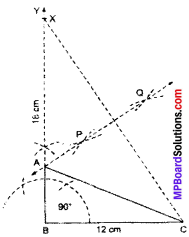# MP Board Class 9th Maths Solutions Chapter 11 Constructions Ex 11.2

## MP Board Class 9th Maths Solutions Chapter 11 Constructions Ex 11.2Question 1.
Construct a triangle ABC in which BC = 7 cm, B = ∠75° and AB + AC = 13 cm.
Solution:
BC = 7 cm
∠B = 15°
AB + BC = 13 cm.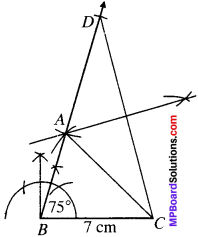Question 2.
Construct a triangle ABC in which BC = 8 cm, ∠B = 45° AB – AC = 3.5 cm.
Solution:
BC = 8 cm
∠B = 45°
AB – AC = 3.5 cm.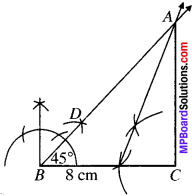Question 3.
Construct a triangle PQR in which QR = 6 cm. ∠Q = 60° and PR – PQ = 2 cm.
Solution:
QR = 6 cm
∠Q =60°
PR – PQ = 2 cm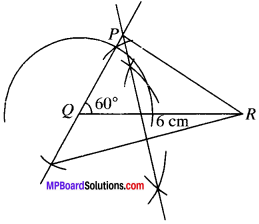Question 4.
Construct a triangle XYZ in which ∠Y = 30°, ∠Z = 90° and XY + YZ + ZX = 11 cm.
Solution:
XY + YZ + ZX = 11 cm
∠Y = 30°
∠Z =90°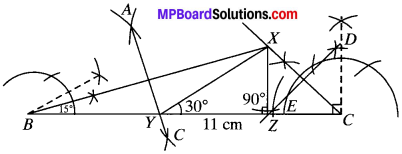1. Draw a line segment BC =11 cm.
2. At B construct an angle of 30° and at C, draw angle of 90°.
3. Bisect these angles. Let the bisectors of these angles intersect atX.
4. Draw perpendicular bisectors AC of BX to intersect BC at Y and DZ of XC to intersect BC at Z.
5. Join XY and XZ. XYZ is the required D.Question 5.
Construct a right triangle whose base is 12 cm and sum of its hypotenuse and other side is 18 cm.
Solution:
Steps of construction:

1. Draw $$\overline { BC }$$ = 12 cm.
2. Construct ∠CBY = 90°.
3. From $$\overline { BY }$$, cut off BX = 18 cm.
4. Join CX.
5. Draw PQ, the perpendicular bisector of CX, such that PQ meets BX at A.
6. JoinAC.

Thus, ABC is the required triangle.